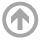Naval Architecture & Ocean Engineering# EN475: Ocean Engineering Mechanics

## Catalog Description

### EN475: Ocean Engineering Mechanics (3-2-4)

This course develops principles of linear wave theory including wavelength, celerity, shoaling, refraction, diffraction, reflection, and breaking. Wave theory is then applied to predict wave forces on fixed and floating structures, with emphasis on vertical walls and circular cylinders. Measurement techniques discussed include measurements of wave height, wave-induced forces, and motions in waves.

### Textbook

• Coastal Engineering Manual (U.S. Army Corps of Engineers)

### Goals

1. Compute basic characteristics of ocean waves through application of linear wave theory.
2. Calculate wave forces on vertical walls and circular cylinders.
3. Estimate the dynamic motions of an ocean structure in waves based on its mass, stiffness, and damping characteristics.
4. Analyze laboratory measurements of wave properties and compare measurements to values computed using linear wave theory.
5. Apply appropriate computer methods, including Microsoft Excel and MATLAB, to compute wave characteristics and/or analyze laboratory data.

### Prerequisites

• EM324
• EN245
• Approval of NAOE Department Chair

### Class Topics

1. Basic Wave Properties and Linear Wave Theory
2. Wave Shoaling and Breaking
3. Wave Refraction and Diffraction
4. Pressures and Forces Due to Standing Waves
5. Wave Drag and Inertia Forces
6. Morison Equation – Forces on Piles
7. Dynamic Motions of Structures in Waves
8. Random Waves